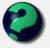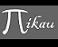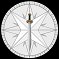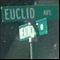# Math Is Fun Forum

Discussion about math, puzzles, games and fun.   Useful symbols: ÷ × ½ √ ∞ ≠ ≤ ≥ ≈ ⇒ ± ∈ Δ θ ∴ ∑ ∫  π  -¹ ² ³ °

You are not logged in.

## #1 2006-01-21 07:25:30

irspow
MemberRegistered: 2005-11-24
Posts: 1,055

### Is there a definition of an integer?

What I am asking might be stupid, but is there a function which would produce only positive integers?

? y = f(x),  where all values of y are positive integers for every real value of x ?

I am at an age where I have forgotten more than I remember, but I still pretend to know it all.

Offline

## #2 2006-01-21 08:00:25

mathsyperson
ModeratorRegistered: 2005-06-22
Posts: 4,900

### Re: Is there a definition of an integer?

You could have y = floor(x) or ceiling(x), but that's kind of cheating.

I can't think of anything else that would do it.

Why did the vector cross the road?
It wanted to be normal.

Offline

## #3 2006-01-21 08:04:54

irspow
MemberRegistered: 2005-11-24
Posts: 1,055

### Re: Is there a definition of an integer?

How would something like that work?  I am working on a proof but I need to relate two functions, one of which only produces integers.

I am at an age where I have forgotten more than I remember, but I still pretend to know it all.

Offline

## #4 2006-01-21 08:10:42

mathsyperson
ModeratorRegistered: 2005-06-22
Posts: 4,900

### Re: Is there a definition of an integer?

I have no idea how floor or ceiling functions work, I've just heard other people talk about them.

I'd assume that floor functions work something like this: y = x - mod(1)x

Why did the vector cross the road?
It wanted to be normal.

Offline

## #5 2006-01-21 08:17:58

irspow
MemberRegistered: 2005-11-24
Posts: 1,055

### Re: Is there a definition of an integer?

Maybe I should cut to the chase.

I came up with a formula that relates the size of an inner sphere with the size of outer spheres which completely surround the inner one as a function of the number of spheres on the perimeter.  In other words,  all outer spheres touching forming a circle around and touching the inner one.

R = r(inner)/r(outer) = [2sin((90n - 180)/n) / sin(360/n)] - 1

I solved this for when the R = 1 then n = 6.

This means that if the inner sphere is the same size as the outer spheres then six will completely envelope the other.

Obviously you can use any number of exterior spheres and produce a ratio, but I want to know when the ratios are integers other than one.  I don't want to spend the rest of my life plugging in different values of n to see if this occurs again.  You see where I am going?

All of the other solutions to this problem give irrational ratios, meaning that they could not be duplicated in the real world.  I just want other real examples of when such a system would work other than having seven equal sized spheres.

I am at an age where I have forgotten more than I remember, but I still pretend to know it all.

Offline

## #6 2006-01-21 08:21:10

mikau
MemberRegistered: 2005-08-22
Posts: 1,504

### Re: Is there a definition of an integer?

Do you mean like could you write a computer program to convert to a positive integer? That would be pretty pointless since, for instance in C++, unsigned integer will do that auotomaticly. As far as a mathematical algorithm to follow, I think thats pretty pointless. A computer program needs a series of steps to follow, and its easy enough to write one, but people are smart enough not to need that. A general definition should do.

A logarithm is just a misspelled algorithm.

Offline

## #7 2006-01-21 08:28:40

irspow
MemberRegistered: 2005-11-24
Posts: 1,055

### Re: Is there a definition of an integer?

Mikau, are you saying that it would be best if I just write a little C++ code to do the job for me?  If that is the case, so be it, I will.  I was just wondering whether or not there was such a function that does the same job.  I am maybe out of touch with the times, but I still enjoy doing such things on paper.

Oh, is there C++ code that mimmicks trigonomic functions?  I will need them if I am going to use the computer to do this one.

Last edited by irspow (2006-01-21 08:31:42)

I am at an age where I have forgotten more than I remember, but I still pretend to know it all.

Offline

## #8 2006-01-21 10:09:33

MathsIsFunRegistered: 2005-01-21
Posts: 7,711

### Re: Is there a definition of an integer?

But before you get too involved,  there are many different programming languages to choose from, each with their advantages and disadvantages.

C++ is elegant and fast, but misses out on its ability to put it live on a website. At the other end, Javascript is a bit "slack", but can be added to a website with great ease. And many choices inbetween !

I see programming as part of Mathematics, but proofs are better done with "pencil and paper" for the simple reason that to use a program as proof would require a complete description of the program, right down to the algorithm used by the sin() function.

Having said that - a program will give you many insights, and allow you to experiment freely with your ideas. And it is fun!

"The physicists defer only to mathematicians, and the mathematicians defer only to God ..."  - Leon M. Lederman

Offline

## #9 2006-01-21 10:17:33

irspow
MemberRegistered: 2005-11-24
Posts: 1,055

### Re: Is there a definition of an integer?

I just mention C++ because I have a compiler for it.  I guess that I'll Google it like you suggest.

By the way, do you think that you can solve the problem above for integer values of R without a computer? Techically, R does not have to be an integer either but rather a real value.

I am at an age where I have forgotten more than I remember, but I still pretend to know it all.

Offline

## #10 2006-01-21 11:57:35

mikau
MemberRegistered: 2005-08-22
Posts: 1,504

### Re: Is there a definition of an integer?

irspow  I think all you have to do is declare a variable

unsigned int x;

If you were to assign a float or decimal value to it, it would automaticly be converted to an int and change the sign if its negative I think.... But I believe, the drawback is it doesn't round. Thus if you assigned a value of 3.9999999999 to an int it would have a value of 3. A rounding function should be relativly easy to write, though.

A logarithm is just a misspelled algorithm.

Offline

## #11 2006-01-21 12:18:57

irspow
MemberRegistered: 2005-11-24
Posts: 1,055

### Re: Is there a definition of an integer?

I think that I have let this topic spiral out of control.  I just want to find when;

n≥3 gives a rational value of R other than when n = 6

R = [2sin((90n - 180)/n) / sin(360/n)] - 1

At n = 6, R = 1,  but most other values take the form of √(4/3) - 1 at n = 3 and √(2) - 1 at n = 4 and so forth.  I'm looking for ratios that I can actually produce in the real world.

From what I have computed so far, there doesn't seem to be another case of a rational R, but I cannot prove it.

I am at an age where I have forgotten more than I remember, but I still pretend to know it all.

Offline

## #12 2006-01-21 12:24:42

God
MemberRegistered: 2005-08-25
Posts: 59

### Re: Is there a definition of an integer?

Here are my functions >:)
y = 1
y = |x| / x
y = sqrt(x^2)

Offline

## #13 2006-01-21 20:06:01

Ricky
ModeratorRegistered: 2005-12-04
Posts: 3,791

### Re: Is there a definition of an integer?

A rounding function should be relativly easy to write, though.

float y;
int x = y + 0.5;

Here are my functions >smile
y = 1
y = |x| / x
y = sqrt(x^2)

Those are not natural numbers.

You want a cool function?  Take the binary value stored in a float, and save that value in an unsigned integer.  This can easily be done with pointers:

#include <iostream>

using namespace std;

int main()
{
float *f = new float;
*f = -5.3;
cout << *((unsigned int *)f) << endl;

delete f;
}

The output is:  3232340378

The thing that's cool about this function is that (on a computer) it's 1-1 and onto.  Of course, in real life it's not because there are a greater amount of fractions (rationals) than there are integers.

Last edited by Ricky (2006-01-21 20:08:21)

"In the real world, this would be a problem.  But in mathematics, we can just define a place where this problem doesn't exist.  So we'll go ahead and do that now..."

Offline

## #14 2006-01-21 20:17:35

Ricky
ModeratorRegistered: 2005-12-04
Posts: 3,791

### Re: Is there a definition of an integer?

R = [2sin((90n - 180)/n) / sin(360/n)] - 1

The problem with a rational test is that 1.1 / 2.4 is rational.  That's 11 / 24.  And there's even more problems with that.  Values such as 1.1 can not be stored entirely in binary.  You can get close, but not exact.  So you can't tell the difference between 1.1 and 1.1000000000000001.  And further more, irrational numbers can't be stored on the computer anyways.  You can have an approximation, but that's it.

I'll think more on this problem tonight, but I believe it can not be solved through a computer.

Last edited by Ricky (2006-01-21 20:18:29)

"In the real world, this would be a problem.  But in mathematics, we can just define a place where this problem doesn't exist.  So we'll go ahead and do that now..."

Offline

## #15 2006-01-21 20:53:40

Ricky
ModeratorRegistered: 2005-12-04
Posts: 3,791

### Re: Is there a definition of an integer?

Oh, is there C++ code that mimmicks trigonomic functions?  I will need them if I am going to use the computer to do this one.

#include <math.h>

http://www.cplusplus.com/ref/cmath/

R = [2sin((90n - 180)/n) / sin(360/n)] - 1

sin(x)/sin(y) is only rational when x = 0 or x = 2Pi*n*y, where n is an integer.  It should be easy to solve from there.

Last edited by Ricky (2006-01-21 20:55:15)

"In the real world, this would be a problem.  But in mathematics, we can just define a place where this problem doesn't exist.  So we'll go ahead and do that now..."

Offline

## #16 2006-01-22 04:03:56

irspow
MemberRegistered: 2005-11-24
Posts: 1,055

### Re: Is there a definition of an integer?

But I got a rational answer when x, as in sin(x)/sin(y), equalled 60 at n=6.  Perhaps your definition of when this function is rational only applies if I was using radians?  This function is predicated on the fact that I am using degrees.  I could adapt it to radians if you feel that this would be beneficial.

I am at an age where I have forgotten more than I remember, but I still pretend to know it all.

Offline

## #17 2006-01-22 04:13:53

Ricky
ModeratorRegistered: 2005-12-04
Posts: 3,791

### Re: Is there a definition of an integer?

People who use degrees are just afriad of Pi.

Heh, just kidding of course.  Degrees works just as well.

sin(x)/sin(y) is only rational when x = 180*n or x = 360*n*y, where n is an integer.

Last edited by Ricky (2006-01-22 04:16:34)

"In the real world, this would be a problem.  But in mathematics, we can just define a place where this problem doesn't exist.  So we'll go ahead and do that now..."

Offline

## #18 2006-01-22 04:23:36

irspow
MemberRegistered: 2005-11-24
Posts: 1,055

### Re: Is there a definition of an integer?

Again, I got a rational R of 1 when x, as defined above, equalled 60, neither of the conditions above when n = 6.

x = 180n = 180*6 ≠ 60
x = 360ny = 360(6)(60) ≠ 60

I am at an age where I have forgotten more than I remember, but I still pretend to know it all.

Offline

## #19 2006-01-22 04:30:21

Ricky
ModeratorRegistered: 2005-12-04
Posts: 3,791

### Re: Is there a definition of an integer?

Scratch all of that.  What you are doing is dividing xπ/yπ, in which both pi's cancle out.  The only time there are no pi's (starting to get a bit hungry), is when x=0 or y=0.  If x=0, xπ/yπ = 0.  If y = 0, you don't have a real value.  So every number is rational.

"In the real world, this would be a problem.  But in mathematics, we can just define a place where this problem doesn't exist.  So we'll go ahead and do that now..."

Offline

## #20 2006-01-22 07:41:26

irspow
MemberRegistered: 2005-11-24
Posts: 1,055

### Re: Is there a definition of an integer?

You are still missing something, Ricky. The function;

R = [2sin((90n - 180)/n) / sin(360/n)] - 1

Does not even have a solution when x = 0 at n = 2.
This makes sense as n is the number of spheres enveloping a single inner sphere.
Actually n has a domain of 3 to ∞.

The successive Rs are:

((2√3)/3)-1,  n=3
√(2) - 1,  n=4
[(√(5) + 1)√(2)] / [√(√(5) + 5)],  n=5
1,  n=6

Essentially, the sin((90n - 180)/n) / sin(360/n) part has to be rational.  You are only multiplying this value by 2 and then subtracting 1 to get R.

I put this function into my TI-89 and then looked at the table.  After scanning up to n = 1000, it seems clear that this function does not produce any further rational ratios.  I guess that I will not be able to prove it mathematically at this time.

The implication is that a 1:1 ratio (exterior spheres radius to the interior sphere radius) is the only time when such an arangement can be made where all spheres touch each other.  This occurs when n = 6, meaning that the seven sphere arrangement is unique.

I am at an age where I have forgotten more than I remember, but I still pretend to know it all.

Offline

## #21 2006-01-22 07:54:26

Ricky
ModeratorRegistered: 2005-12-04
Posts: 3,791

### Re: Is there a definition of an integer?

Ah, you're right.  I completely forgot about roots.

"In the real world, this would be a problem.  But in mathematics, we can just define a place where this problem doesn't exist.  So we'll go ahead and do that now..."

Offline

## #22 2006-01-22 08:08:08

irspow
MemberRegistered: 2005-11-24
Posts: 1,055

### Re: Is there a definition of an integer?

I guess this case is closed.  Now I have to find what the implications of this arrangement have on spheres occupying space in general.

One that I have found already is that this pattern is repeated again and again when maximizing the number of spheres within an enclosed container.  Any other arrangement leads to more wasted volume.  I guess that this arrangement also suggests a point of stable equilibrium for potential energy.

Well, thanks to everyone who I pestered on this topic.  I appreciate all of the input, as even the now irrelevant posts did serve to drive me closer to the solution that I was looking for.

I am at an age where I have forgotten more than I remember, but I still pretend to know it all.

Offline